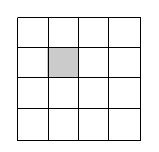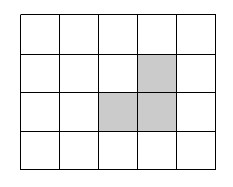### Home > MC1 > Chapter 10 > Lesson 10.1.3 > Problem10-34

10-34.

Find the area of each shaded portion below, if the entire square represents one whole. Can you find the answer in more than one way?

1.2.You should first try to find the area of the entire rectangle.
Remember, the area of something is the total of the one unit squares that fit inside of a shape.
Do you remember the two ways to find the area of something?

If the square has $16$ squares inside of it, and the shaded portion is just one square, can you determine the shaded area?

The shaded area is $\frac{1}{16}$.

Can you think of multiple ways to represent this?

Gilly said that this area is the same as the length times the width of the portion $\left(\frac{1}{4} \cdot \frac{1}{4}\right)$.